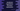# JavaScript logarithmic functions## JavaScript logarithmic functions :

JavaScript Math is a built-in object that has different mathematical constants and functions. It has a couple of different functions and constants. In this post, I will show you all the logarithmic functions with examples.

### Logarithmic functions defined in Math :

Following are the logarithmic functions defined in JavaScript Math :

``````1. Math.log()
2. Math.log10()
3. Math.log1p()
4. Math.log2()``````

## 1. Math.log() :

Math.log() function returns the base e logarithm or natural logarithm of a number. It takes one number as its argument. It returns NaN for negative numbers. For example :

``````console.log(Math.log(2));
console.log(Math.log(1));
console.log(Math.log(0));
console.log(Math.log(10));
console.log(Math.log(-9));``````

It will print :

``````0.6931471805599453
0
-Infinity
2.302585092994046
NaN``````

## 2. Math.log10() :

Math.log10() function returns the base 10 logarithm of a number. It takes one number as its argument. For negative number, it returns NaN.

``````console.log(Math.log10(10));
console.log(Math.log10(100));
console.log(Math.log10(0));
console.log(Math.log10(1));
console.log(Math.log10(-9));``````

Output :

``````1
2
-Infinity
0
NaN``````

## 3. Math.log1p() :

Math.log1p() function returns the natural logarithm or base e logarithm of 1 + one number. It is similar to Math.log(). For negative numbers, it returns NaN. For example :

``````console.log(Math.log1p(0));
console.log(Math.log1p(1));
console.log(Math.log1p(-10));
console.log(Math.log1p(2));``````

It will print :

``````0
0.6931471805599453
NaN
1.0986122886681096``````

## 4. Math.log2() :

Math.log2() function returns the base 2 logarithm of a number. It returns NaN for negative numbers. For example :

``````console.log(Math.log2(0));
console.log(Math.log2(1));
console.log(Math.log2(-10));
console.log(Math.log2(4));``````

It will print :

``````-Infinity
0
NaN
2``````

It is equivalent to log(num)/log(2). For example :

``````console.log(`\${Math.log2(0)}, \${Math.log(0)/Math.log(2)}`);
console.log(`\${Math.log2(4)}, \${Math.log(4)/Math.log(2)}`);
console.log(`\${Math.log2(10)}, \${Math.log(10)/Math.log(2)}`);
console.log(`\${Math.log2(-4)}, \${Math.log(-4)/Math.log(2)}`);``````

It will print :

``````-Infinity, -Infinity
2, 2
3.321928094887362, 3.3219280948873626
NaN, NaN``````

You can see here that both return the same result.